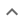•# Factoring multivariable polynomials

We think you wrote:

###This solution deals with factoring multivariable polynomials.

Solution found(x+8y)^2

## Step by Step Solution## Step  1  :

#### Equation at the end of step  1  :

  ((x2) +  16xy) +  26y2


## Step  2  :

#### Trying to factor a multi variable polynomial :

Factoring    x2 + 16xy + 64y2

Try to factor this multi-variable trinomial using trial and error

Found a factorization  :  (x + 8y)•(x + 8y)

#### Detecting a perfect square :

x2  +16xy  +64y2  is a perfect square

It factors into  (x+8y)•(x+8y)
which is another way of writing  (x+8y)2

How to recognize a perfect square trinomial:

• It has three terms

• Two of its terms are perfect squares themselves

• The remaining term is twice the product of the square roots of the other two terms

## Final result :

  (x + 8y)2